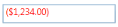# Culture and Formatting in WPF Currency TextBox

21 May 20216 minutes to read

Value of `CurrencyTextBox` can be formatted in following ways:

• Culture
• NumberFormatInfo
• Dedicated properties (CurrencyGroupSeparator, CurrencyGroupSizes, CurrencyDecimalDigits, CurrencyDecimalSeparator)

## Culture based formatting

The CurrencyTextBox provides support for globalization by using the Culture property. The `Culture` property is used to format the decimal separator and group separator of the `CurrencyTextBox` value based on the respective culture.

``<syncfusion:CurrencyTextBox x:Name="currencyTextBox" Height="25" Width="150" Culture="fr-FR" Value="1234567"/>``
``````CurrencyTextBox currencyTextBox = new CurrencyTextBox();
currencyTextBox.Width = 100;
currencyTextBox.Height = 25;
currencyTextBox.Value = 1234567;
currencyTextBox.Culture = new CultureInfo("fr-FR");``````

By default the US culture uses “,” as the `CurrencyGroupSeparator` “\$” as `CurrencySymbol` and “.” as the `CurrencyDecimalSeparator` where as the France culture uses “Space” as the `CurrencyGroupSeparator`, “€” as `CurrencySymbol` and “,” as the `CurrencyDecimalSeparator`.

Default Culture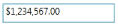France Culture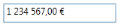## NumberFormatInfo based formatting

The number formatting of `CurrencyTextBox` can be customized by setting NumberFormat property.

``````<syncfusion:CurrencyTextBox x:Name="currencyTextBox" Height="25" Width="150"  Value="1234567">
<syncfusion:CurrencyTextBox.NumberFormat >
<numberformat:NumberFormatInfo CurrencyGroupSeparator="/"
CurrencyDecimalDigits="4"
CurrencyDecimalSeparator="*"
CurrencySymbol="\$"/>
</syncfusion:CurrencyTextBox.NumberFormat>
</syncfusion:CurrencyTextBox>``````
``````CurrencyTextBox currencyTextBox = new CurrencyTextBox();
currencyTextBox.Width = 150;
currencyTextBox.Height = 25;
currencyTextBox.Value = 1234567;
currencyTextBox.NumberFormat = new NumberFormatInfo()
{
CurrencyGroupSeparator = "/",
CurrencyDecimalDigits = 4,
CurrencyDecimalSeparator = "*",
CurrencySymbol = "\$"
};``````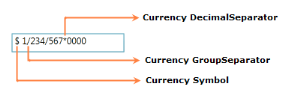The following code illustrate how to set currency group size by using the `NumberFormat` property.

``````CurrencyTextBox currencyTextBox = new CurrencyTextBox();
currencyTextBox.Width = 150;
currencyTextBox.Height = 25;
currencyTextBox.Value = 123456789;
currencyTextBox.NumberFormat = new NumberFormatInfo()
{
CurrencySymbol = "&",
CurrencyDecimalDigits = 4,
CurrencyGroupSeparator = "/",
CurrencyDecimalSeparator = "*",

// Adding the currency group size via NumberFormat property.
CurrencyGroupSizes = new int[] { 2, 3, 4 }
};``````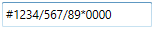## Formatting with dedicated properties

The number formatting of `CurrencyTextBox` can also be customized by setting the CurrencyGroupSeparator, CurrencyGroupSizes, CurrencyDecimalDigits and CurrencyDecimalSeparator, CurrencySymbol, CurrencyNegativePattern, and CurrencyPositivePattern properties of CurrencyTextBox. You can show the group separator by enable the GroupSeperatorEnabled property to `true`.

The following code illustrate how to format using the `CurrencyDecimalSeparator`, `CurrencyDecimalDigits`, `CurrencyGroupSeparator`, `CurrencyGroupSizes` property of the `CurrencyTextBox`.

``````CurrencyTextBox currencyTextBox = new CurrencyTextBox();
currencyTextBox.Width = 150;
currencyTextBox.Height = 25;
currencyTextBox.Value = 123456789;
currencyTextBox.CurrencySymbol = "#";
currencyTextBox.CurrencyDecimalDigits = 4;
currencyTextBox.GroupSeperatorEnabled = true;
currencyTextBox.CurrencyGroupSeparator = "/";
currencyTextBox.CurrencyDecimalSeparator = "*";

// Adding the currency group size via CurrencyGroupSizes property.
currencyTextBox.CurrencyGroupSizes = new Int32Collection() { 4, 3, 2 };``````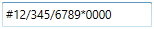NOTE

When you use both the `NumberFormat` and the dedicated properties (`CurrencyGroupSeparator`, `CurrencySymbol`, `CurrencyDecimalDigits`, `CurrencyDecimalSeparator` and `CurrencyGroupSizes`) to format the value of `CurrencyTextBox`, the `CurrencyGroupSeparator`and `CurrencyGroupSizes` properties have higher priority.

NOTE

When you use both `NumberFormat` and `Culture`, the `NumberFormat` will have a higher priority.

### Positive Value Pattern

You can use the CurrencyPositivePattern property to customize the location of the currency symbol and the positive currency values. In the table below,”\$” denotes the symbol of the currency, and “n” denotes the number.

CurrencyPositivePattern table

Value Associated Pattern
0 \$n
1 n\$
2 \$ n
3 n \$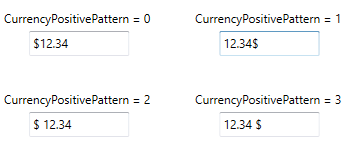``<syncfusion:CurrencyTextBox x:Name="currencyTextBox" Height="25" Width="150" Value="1234" CurrencyPositivePattern="3"/>``
``````CurrencyTextBox currencyTextBox = new CurrencyTextBox();
currencyTextBox.Width = 150;
currencyTextBox.Height = 25;
currencyTextBox.Value = 1234;
currencyTextBox.CurrencyPositivePattern = 3;``````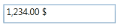### Negative Value Pattern

You can use the CurrencyNegativePattern property to customize the location of the currency symbol and the negative currency values. In the table below,”\$” denotes the symbol of the currency, and “n” denotes the number.

CurrencyNegativePattern table

Value Associated Pattern
0 (\$n)
1 -\$n
2 \$-n
3 \$n-
4 (n\$)
5 -n\$
6 n-\$
7 n\$-
8 -n \$
9 -\$ n
10 n \$-
11 \$ n-
12 \$ -n
13 n- \$
14 (\$ n)
15 (n \$)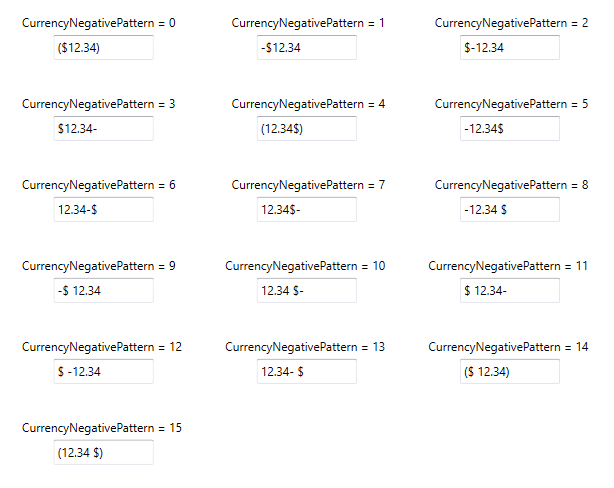``<syncfusion:CurrencyTextBox x:Name="currencyTextBox" Height="25" Width="150" Value="-1234" CurrencyNegativePattern="0"/>``
``````CurrencyTextBox currencyTextBox = new CurrencyTextBox();
currencyTextBox.Width = 150;
currencyTextBox.Height = 25;
currencyTextBox.Value = -1234;
currencyTextBox.CurrencyNegativePattern = 0;``````